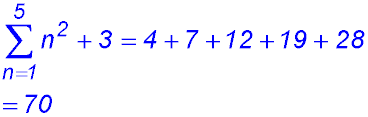Dr. J's Maths.com
Where the techniques of Maths
are explained in simple terms.

Sequences & Series - Sigma notation.
Test Yourself 1 - Solutions.

 General interpretation. 1.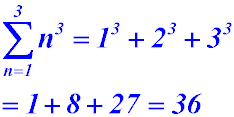2.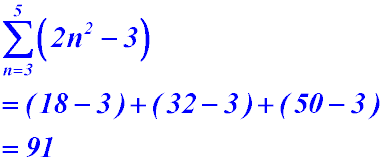3.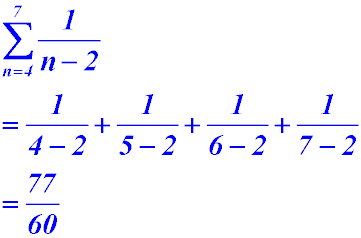4. 5.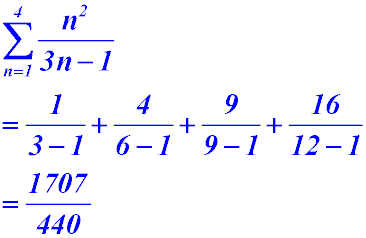6.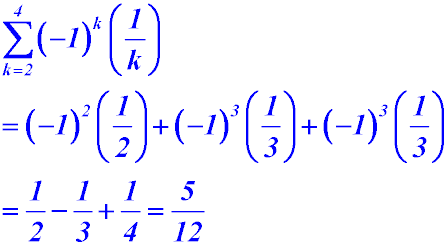Use with arithmetic series. (i)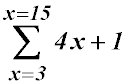is an arithmetic series because the model is a linear equation and the common difference is the gradient (i.e. 4). OR The series is 13 + 17 + 21 + ... 61 which has a common difference of 4. So the series is arithmetic. (ii) Hence evaluate this summation. T1 = 13; n = (15-2); T13 = 61.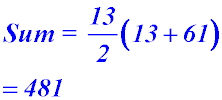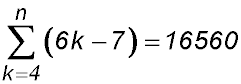When k = 4, the term is 17 and we have a common difference of 6 (coefficient of the expression after sigma sign).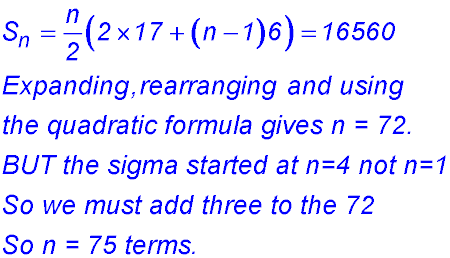Use with geometric series.16.